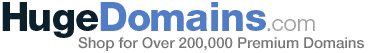# Matlab - The Fourier Series

• MATLAB
I have some problems on 'for' and 'while' loop so I could not write a matlab program for this question;.

Well, how can I start?

First of all, I assume you are writing a .m script file i.e. you start off with:
Code:
function y = f(x)

%%The loop would look like:

y=0.0;

for n = 1:50

y = y + 2(-1)^(n+1) ( (pi^2)/n - (6/(n^3)) sin(n x);

end

%%After which you could write

x = 1.0 : 0.1 : 2*pi
y = f(x);
plot(y,x)

Oh, I got the point! Thanks for replying..

Hi, I am trying to plot a fourier series in matlab for the function

x(t)

= 0 when -pi < wt < 0
= (4/pi)*wt when 0 < wt < pi/2
= 2 when pi < wt < 2*pi

I know that a0 = 3/2, an = (1/pi)*(4/(pi*i^2))*(cos((pi*i/2)-1)), and bn = (1/pi)*((4/(pi*i^2))*(sin(pi*i/2-1))-2/pi*(cos(pi*i)))

so far I have the code as:

N = 11;
x = [0:100]/100;
f = zeros(1,101)*1/2;
for i = 1:2:N
a = (1/pi)*(4/(pi*i^2))*(cos((pi*i/2)-1));
b = (1/pi)*((4/(pi*i^2))*(sin(pi*i/2-1))-2/pi*(cos(pi*i)));
f = f + a*cos(i*x)+ b*sin(i*x);
end
f = .75 + f;

plot(x,f);

any help?? I tried tweaking the window so that it would plot the entire period, but it's not coming out correctly. any help??

Hi, this is the code I did for my first assignment on fourier series. It might be considered "cheating" in terms of programming since I used the Matlab's symbolic method to do all the grunt work. I figured if Matlab offered such a nice/easy feature then I might as well use it, lol :P

For a square wave:
$$V(x)=\left\{\begin{matrix} 1 & 0<x<\frac{L}{2}\\ -1 & \frac{L}{2}<x<L \end{matrix}\right.$$

Fourier Series
$$V_{N}(x)=\sum_{n=0}^{N}a_{n}\sqrt{\frac{2}{L}}cos(\frac{n2\pi x}{L}) + \sum_{n=1}^{N}b_{n}\sqrt{\frac{2}{L}}sin(\frac{n2\pi x}{L})$$

where
$$a_{n} = \frac{2L}{n\pi}\sqrt{\frac{2}{L}}sin^2(\frac{n\pi}{2})sin(n\pi)$$

$$b_{n} = -\frac{2L}{n\pi}\sqrt{\frac{2}{L}}sin^2(\frac{n\pi}{2})cos(n\pi)$$

One can change the summation limit N in the code below by changing the value 20, the last parameter in the function

V_x = symsum(....., n, 1, 20);

Code:
L = 8;
syms n x;

a_n = sqrt(2/L)*( (2*L)/(n*pi) )*( (sin((n*pi)/2))^2 )*sin(n*pi); %fourier coefficient a_n
b_n = -sqrt(2/L)*( (2*L)/(n*pi) )*( (sin((n*pi)/2))^2 )*cos(n*pi); %fourier coefficient b_n
x = 0:0.2:8; %range
V_x = symsum( a_n*sqrt(2/L)*cos( (n*2*pi*x)/L ) + b_n*sqrt(2/L)*sin( (n*2*pi*x)/L ), n, 1, 9 ); %fourier series

plot(x, V_x);
grid on;
axis([ 0 8 -1.4 1.4]);
hold on;

Last edited: